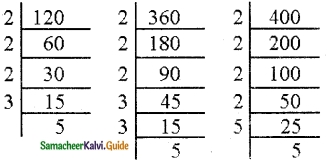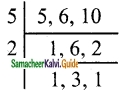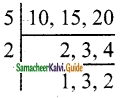Students can download Maths Chapter 1 Numbers Ex 1.3 Questions and Answers, Notes, Samacheer Kalvi 6th Maths Guide Pdf helps you to revise the complete Tamilnadu State Board New Syllabus, helps students complete homework assignments and to score high marks in board exams.

## Tamilnadu Samacheer Kalvi 6th Maths Solutions Term 2 Chapter 1 Numbers Ex 1.3

Miscellaneous Practice Problems

Question 1.
Every even number greater than 2 can be expressed as the sum of two prime numbers. Verify this statement for every even number upto 16.
Solution:
Even numbers greater then 2 upto 16 are 4, 6, 8, 10, 12, 14 and 16
4 = 2 + 2
6 = 3 + 3
8 = 3 + 5
10 = 3 + 7 (or) 5 + 5
12 = 5 + 7
14 = 7 + 7 (or) 3 + 11
16 = 5 + 11 (or) 3 + 13Question 2.
Is 173 a prime? Why?
Solution:
Yes, 173 is a prime. Because it has only 1 and itself as factors.

Question 3.
For which of the numbers, from n = 2 to 8.
Is 2n – 1 a prime?
Solution:
n = 2 ⇒ 2n – 1 = 2 × 2 – 1
= 4 – 1
= 3 (prime)
n = 3 ⇒ 2n – 1 = 2 × 3 – 1
= 6 – 1
= 5 (prime)
n = 4 ⇒ 2n – 1 = 2 × 4 – 1
= 8 – 1
= 7 (prime)
n = 5 ⇒ 2n – 1 = 2 × 5 – 1
= 10 – 1
= 9 (Not prime)
n = 6 ⇒ 2n – 1 = 2 × 6 – 1
= 12 – 1
= 11 (prime)
n = 7 ⇒ 2n – 1 = 2 × 7 – 1
= 14 – 1
= 13
n = 8 ⇒ 2n – 1 = 2 × 8 – 1
= 16 – 1
= 15 (Not prime)Question 4.
(a) A number is divisible by 9 if it is divisible by 3.
(b) A number is divisible by 6 if it is divisible by 12.
Solution:
(i) False, 42 is divisible by 3 but it is not divisible by 9
(ii) True, 36 is divisible by 12. Also divisible by 6.

Question 5.
Find A as required
(i) The greatest 2 digit number 9 A is divisible by 2.
(ii) The least number 567A is divisible by 3.
(iii) The greatest 3 digit number 9A6 is divisible by 6.
(iv) The number A08 is divisible by 4 and 9.
(v) The number 225A85 is divisible by 11.
Solution:
(i) 98 A = 8
(ii) 5670 A = 0
(iii) 996 A = 9
(iv) 108 A = 1
(v) 225885 A = 8Question 6.
Numbers divisible by 4 and 6 are divisible by 24. Verify this statement and support your answer with an example.
Solution:
False 12 is divisible by both 4 and 6. But not divisible by 24

Question 7.
The sum of any two successive odd numbers is always divisible by 4. Justify this statement with an example.
Solution:
True 3 + 5 = 8 is divisible by 4.

Question 8.
Find the length of the longest rope that can be used to measure exactly the ropes of length 1 m 20cm, 3m 60 cm and 4 m.
Solution:
1 m 20 cm = 120 cm
3 m 60 cm = 360 cm
4 m = 400 cm
This is a HCF related problem. So, we need to find the HCF of 120,360 and 400.120 = 2 × 2 × 2 × 3 × 5
360 = 2 × 2 × 2 × 3 × 3 × 5
400 = 2 × 2 × 2 × 2 × 5 × 5
HCF = 2 × 2 × 2 × 5 = 40
The length of the longest rope = 40 cmChallenge Problems

Question 9.
The sum of three prime numbers is 80. The difference between the two of them is 4. Find the numbers.
Solution:
Given the sum of three prime numbers is 80
The numbers will be one or two-digit prime numbers. Also one of them is 2
Sum of the remaining 2 numbers = 78 [∵ one number must be even]
Also their difference = 4 (given) [Otherwise sum of three odd numbers is odd]
The numbers will be 37 and 41
The required numbers are 2, 37, 41

Question 10.
Find the sum of all the prime numbers between 10 and 20 and check whether that sum is divisible by all the single-digit numbers.
Solution:
Prime numbers between 10 and 20 are 11, 13, 17 and 19
Sum = 11 + 13 + 17 + 19 = 60
60 is divisible by 1, 2, 3, 4, 5 and 6.

Question 11.
Find the smallest number which is exactly divisible by all the numbers from 1 to 9.
Solution:
2520Question 12.
The product of any three consecutive numbers is always divisible by 6. Justify this statement with an example.
Solution:
Yes. Because one of every two consecutive integers is even and so the product of three consecutive integers is even and divisible by 2.
Also one of every 3 consecutive integers is divisible by 3.
Product of any three consecutive integers is divisible by 6.
Example: 5 × 6 × 7

Question 13.
Malarvizhi, Karthiga, and Anjali are friends and natives of the same village. They work in different places. Malarvizhi comes to her home once in 5 days. Similarly, Karthiga and Anjali come to their homes once in 6 days and 10 days respectively, Assuming that they met each other on the 1st of October, when will all the three meet again?
Solution:
This is an LCM related problem. So, we need to find the LCM of 5, 6, and 10.LCM = 5 × 2 × 1 × 3 × 1
= 30
All the three will meet again once in 30 days.

Question 14.
In an apartment consisting of 108 floors, two lifts A & B starting from the ground floor, stop at every 3rd and 5th floors respectively. On which floors, will both of them stop together?
Solution:
LCM of 3 and 5 = 3 × 5 = 15
The lifts stop together at floors 15, 30, 45, 60, 75, 90, and 105.Question 15.
The product of 2 two-digit numbers is 300 and their HCF is 5. What are the numbers?
Solution:
15 × 20 = 300
HCF of 15 and 20 is 5
The numbers are 15 and 20

Question 16.
Find whether the number 564872 is divisible by 88. (use of the test of divisibility rule for 8 and 11 will help)
Solution:
564872 Divisibility by 8
564872 It is divisible by 8
Divisibility by 11
5 + 4 + 7 = 16
6 + 8 + 2 = 16
16 – 16 = 0
It is divisible by both 8 and 11 and hence divisible by 88.Question 17.
Wilson, Mathan, and Guna can complete one round of a circular track in 10, 15, and 20 minutes respectively. If they start together from at the starting point of 7 am, at what time will they meet together again at the same starting point?
Solution:
This is an LCM related problem. So, we need to find the LCM of 10, 15, and 20.LCM = 5 × 2 × 1 × 3 × 2 = 60 min
They will meet together again after 60 minutes.
ie. 7.am + 60 minutes = 8 am.